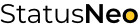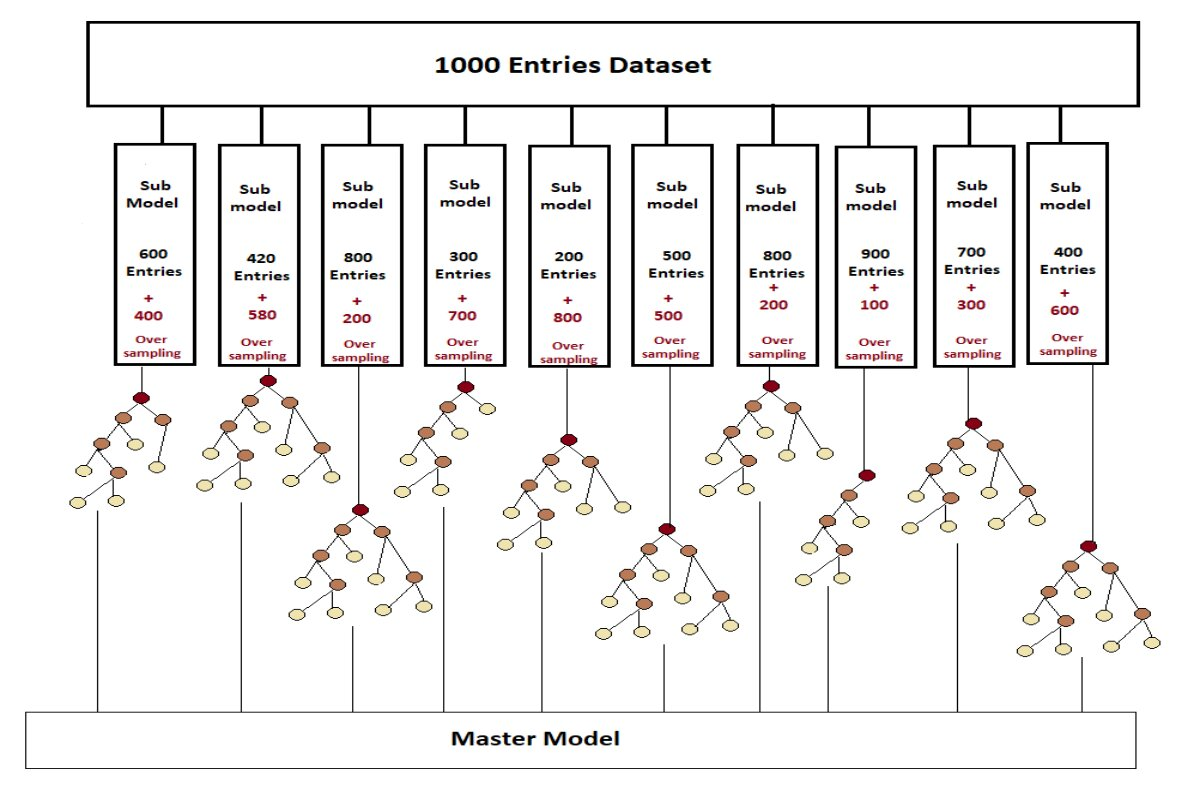WE ARE EXPERTS IN TECHNOLOGY

## Let’s Work Together# StatusNeo## Nature’s Wisdom Unleashed: How the Arboreal Ensemble Revolutionizes Machine Learning

Random forest is a type of supervised machine learning that is used for classification as well as regression. Random forest is a learning method that makes decisions by combining the opinions of many trees. This is nothing but the forest, and each tree works on a random subset of the data, this is known as the random distribution of data. In this way, we can assume what a random forest is.

We know the decision tree (if not, then click here). A tree created on the if-else question-answer. But we often face the situation of overfitting with decision trees because models learn from training data very efficiently but are unable to apply that learning to testing. Since overfit occurs several times.

To avoid the overfitting issue, we can use hyperparameter tuning, pruning techniques, or an advanced algorithm of the decision tree. And Random Forest is one of the advanced algorithm of decision trees, which avoids the overfitting issue by creating multiple trees.

Decision trees built on only one tree, and all decisions take place based on that single tree. We have to accept the predictions because there is no other predictions to compare the answers with, and that’s why there are high possibilities of model overfitting. That means accuracy is much better while training the model and much lower when testing. But in random forests, which build multiple sub-trees and compare all model’s predictions with each other, while comparing if there is a classification problem, the model uses voting (We have two types of voting: Hard vote and Soft vote. Hard vote takes place by majority, and a soft vote takes place by finding probability), and if there is a regression-related problem, then the mean of all sub model predictions is the answer to that particular input.

## Let’s understand it with a simple example:

Suppose there is a company, and a decision has to be made to launch a new product. Now suppose that in that company, if the only company owner is taking all the decisions, then there is no option to avoid that decision. After a few days, we will know whether that decision was right or not. This is an example of a decision tree. But suppose there is a team of some people to take any decision in that company, then what will happen now here is that everyone will discuss the decision with their own experience, and the decision that was made by the maximum number of people will be sealed. This is an example of a random forest. Because what is happening here is that the decision is verified by the maximum peoples, and finally that decision is given the correct contract.

Similarly random forest works in this manner, instead of single tree, the decision will takes place by multiple sub models. This increases the reliability, and the earlier problem of overfitting the decision tree disappears.

## How does random forest work?

As we know, the random forest is the advance algorithm of the decision tree, since all operations related to building a tree are similar to the decision tree. If you are unaware of the workings of the decision tree, then click here.

## Random forest use Ensemble techniques.

Ensemble techniques are methods that combine the predictions of multiple models (sub models, weak learners) to improve overall performance and make more accurate predictions. The main aim of ensemble techniques is to combine the opinions of different models to get a better and more robust result than using any single model alone. There are several types of ensemble techniques, but two are the most popular:

### Bagging:

Bagging stands for bootstrap Aggregating. It creates multiple copies of the same model, each of which is going to train on a different random subset of the original data. The subsets are created by random sampling of the data, which means some data points may appear in multiple subsets while others may not appear at all. Each model in the ensemble learns from its subset and makes predictions accordingly.

### Boosting:

Boosting is another type of ensemble technique where models are trained sequentially, and each new model tries to correct the errors made by the previous ones by getting a list of misclassified and truly classified data. Unlike bagging, boosting focuses more on difficult patterns in data points that were misclassified in the previous sub model, which gives hints to focus on such areas regardless of whether the previous one performed well. This way, it can gradually improve the overall prediction accuracy.

## Now let’s understand step by step with an image.

Suppose there is a dataset with 1000 entries and 10 sub models in this random forest. Now, while working on this in the ensembling method, the data will be randomly distributed among these ten sub models.

Since the data is randomly distributed among these sub-models, there is no certainty as to how much data will go into each sub model, so some may have the maximum amount of data and some less. But one clear thing is that the more data a sub model contains, the more reliable the performance of that model is, and the less reliable the model is.

Now suppose the data is distributed as given above. Now note one thing here: our original dataset had 1000 entries, but now these sub model has not reached the number of 1000, so now complete this number, the required amount of data set is done by oversampling.

A total of 10 sub-models are now composed of the original amount of data. Now the next procedure to make the tree is completely similar to the decision tree. That means selecting the best root node using entropy or the Gini index and growing the tree further in the same manner.

Now a total of 10 trees with 10 models have been created. Until now, a single tree was formed in the decision tree, and we used to trust its prediction, but now not one but numerous trees are formed in the random forest, due to which the prediction of the tree whose prediction will be repeated more often will be selected if there is a classification. And if there is regression, then the mean of the answers from all those submodels will be calculated, and this will be the mean result. Now that there are 10 auspicious models, all these answers will be combined, and a master model will be made. And this method of combining is called aggregating.

1. It reduces the overfitting of Decision Tree and Helps to Improve
accuracy
2. Use for CLassification as well as Regression
3. Feature Scaling is not Required
4. Not Impacted by Outliers